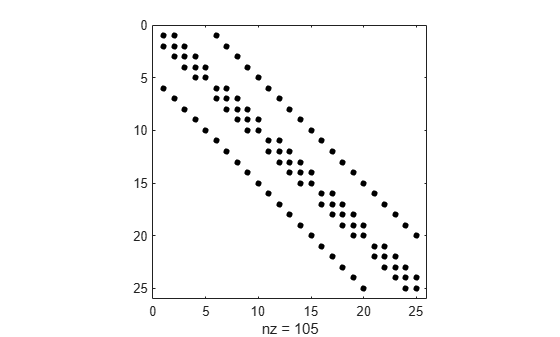# kron

Kronecker tensor product

## Syntax

``K = kron(A,B)``

## Description

example

````K = kron(A,B)` returns the Kronecker tensor product of matrices `A` and `B`. If `A` is an `m`-by-`n` matrix and `B` is a `p`-by-`q` matrix, then `kron(A,B)` is an `m*p`-by-`n*q` matrix formed by taking all possible products between the elements of `A` and the matrix `B`.```

## Examples

collapse all

Create a block diagonal matrix.

Create a 4-by-4 identity matrix and a 2-by-2 matrix that you want to be repeated along the diagonal.

```A = eye(4); B = [1 -1;-1 1];```

Use `kron` to find the Kronecker tensor product.

`K = kron(A,B)`
```K = 8×8 1 -1 0 0 0 0 0 0 -1 1 0 0 0 0 0 0 0 0 1 -1 0 0 0 0 0 0 -1 1 0 0 0 0 0 0 0 0 1 -1 0 0 0 0 0 0 -1 1 0 0 0 0 0 0 0 0 1 -1 0 0 0 0 0 0 -1 1 ```

The result is an 8-by-8 block diagonal matrix.

Expand the size of a matrix by repeating elements.

Create a 2-by-2 matrix of ones and a 2-by-3 matrix whose elements you want to repeat.

```A = [1 2 3; 4 5 6]; B = ones(2);```

Calculate the Kronecker tensor product using `kron`.

`K = kron(A,B)`
```K = 4×6 1 1 2 2 3 3 1 1 2 2 3 3 4 4 5 5 6 6 4 4 5 5 6 6 ```

The result is a 4-by-6 block matrix.

This example visualizes a sparse Laplacian operator matrix.

The matrix representation of the discrete Laplacian operator on a two-dimensional, `n`-by- `n` grid is a `n*n`-by- `n*n` sparse matrix. There are at most five nonzero elements in each row or column. You can generate the matrix as the Kronecker product of one-dimensional difference operators. In this example `n = 5`.

```n = 5; I = speye(n,n); E = sparse(2:n,1:n-1,1,n,n); D = E+E'-2*I; A = kron(D,I)+kron(I,D);```

Visualize the sparsity pattern with `spy`.

`spy(A,'k')`## Input Arguments

collapse all

Input matrices, specified as scalars, vectors, or matrices. If either `A` or `B` is sparse, then `kron` multiplies only nonzero elements and the result is also sparse.

Data Types: `single` | `double` | `int8` | `int16` | `int32` | `int64` | `uint8` | `uint16` | `uint32` | `uint64` | `logical`
Complex Number Support: Yes

collapse all

### Kronecker Tensor Product

If `A` is an `m`-by-`n` matrix and `B` is a `p`-by-`q` matrix, then the Kronecker tensor product of `A` and `B` is a large matrix formed by multiplying `B` by each element of `A`

`$A\otimes B=\left[\begin{array}{ccc}\begin{array}{cc}{a}_{11}B& {a}_{12}B\end{array}& \cdots & {a}_{1n}B\\ \begin{array}{cc}\begin{array}{c}{a}_{21}B\\ ⋮\end{array}& \begin{array}{c}{a}_{22}B\\ ⋮\end{array}\end{array}& \begin{array}{c}\cdots \\ \ddots \end{array}& \begin{array}{c}{a}_{2n}B\\ ⋮\end{array}\\ \begin{array}{cc}{a}_{m1}B& {a}_{m2}B\end{array}& \cdots & {a}_{mn}B\end{array}\right].$`

For example, two simple 2-by-2 matrices produce

`$\begin{array}{l}A=\left[\begin{array}{cc}1& -2\\ -1& 0\end{array}\right],\text{\hspace{0.17em}}\text{\hspace{0.17em}}\text{\hspace{0.17em}}\text{\hspace{0.17em}}B=\left[\begin{array}{cc}4& -3\\ 2& 3\end{array}\right]\\ \\ A\otimes B=\left[\begin{array}{cccc}1\text{\hspace{0.17em}}·\text{\hspace{0.17em}}4& 1\text{\hspace{0.17em}}·\text{\hspace{0.17em}}-3& -2\text{\hspace{0.17em}}·\text{\hspace{0.17em}}4& -2\text{\hspace{0.17em}}·\text{\hspace{0.17em}}-3\\ 1\text{\hspace{0.17em}}·\text{\hspace{0.17em}}2& 1\text{\hspace{0.17em}}·\text{\hspace{0.17em}}3& -2\text{\hspace{0.17em}}·\text{\hspace{0.17em}}2& -2\text{\hspace{0.17em}}·\text{\hspace{0.17em}}3\\ -1\text{\hspace{0.17em}}·\text{\hspace{0.17em}}4& -1\text{\hspace{0.17em}}·\text{\hspace{0.17em}}-3& 0\text{\hspace{0.17em}}·\text{\hspace{0.17em}}4& 0\text{\hspace{0.17em}}·\text{\hspace{0.17em}}-3\\ -1\text{\hspace{0.17em}}·\text{\hspace{0.17em}}2& -1\text{\hspace{0.17em}}·\text{\hspace{0.17em}}3& 0\text{\hspace{0.17em}}·\text{\hspace{0.17em}}2& 0\text{\hspace{0.17em}}·\text{\hspace{0.17em}}3\end{array}\right]=\left[\begin{array}{cccc}4& -3& -8& 6\\ 2& 3& -4& -6\\ -4& 3& 0& 0\\ -2& -3& 0& 0\end{array}\right].\end{array}$`